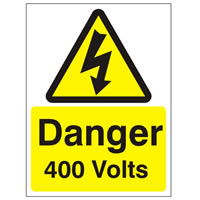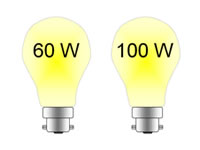# Difference between Volts and Watts

##### Key difference: Volts and Watts are both units of measurement. Volt (V) is the SI derived unit of measurement for voltage. Volts are measured using a voltmeter. Watts (W), on the other hand, is the SI derived unit of measurement for power. The watt is named after the Scottish engineer James Watt. SI stands for the International System of Units, which are the internationally accepted and used units of measurement.Volts and Watts are both units of measurement. Volt (V) is the SI derived unit of measurement for voltage. Volts are measured using a voltmeter. Watts (W), on the other hand, is the SI derived unit of measurement for power. The watt is named after the Scottish engineer James Watt. SI stands for the International System of Units, which are the internationally accepted and used units of measurement.

A single volt is defined as “the difference in electric potential across a wire when an electric current of one ampere dissipates one watt of power,” essentially voltage. Voltage is the electric potential between two different points. It could also be used to refer to the difference in electric potential energy of a unit test charge transported between two points. A voltage may represent a source of energy or it could represent lost, used or stored energy.

Voltage is the pressure of pushing electrons along in a circuit. Two paths require voltage in order for a current to pass through them. Voltage is also the total energy required to move a small electric charge between two points. Voltage is defined in such a way that negatively charged objects are pulled towards higher voltages, while positively charged voltages are pulled towards lower voltages.A single watt is defined as “the rate at which work is done when an object's velocity is held constant at one meter per second against constant opposing force of one newton.” In terms of electromagnetism, one watt is “the rate at which work is done when one ampere (A) of current flows through an electrical potential difference of one volt (V).” Essentially, watt is the measure of power.

Power is the rate at which energy is used or consumed. It is the rate at which the work is accomplished. Essentially power is the energy used over time. (P = J/s). For example, if one does 100 joules of work in one second using 100 joules of energy, the power is 100 watts. Watt is equal to one joule per second. Another unit for power is the kilowatt (kW), where one kW is equal to 1000 watts.

Image Courtesy: nelstarsigns.co.uk, passion4science.wordpress.com